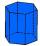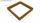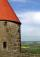# Planimetrics - math word problems

Study plane measurements, including angles, distances, and areas. In other words - measurement and calculation of shapes in the plane. Perimeter and area of plane shapes.

#### Number of problems found: 1824

• Lateral surface areaThe ratio of the area of the base of the rotary cone to its lateral surface area is 3: 5. Calculate the surface and volume of the cone, if its height v = 4 cm.
• Paper boxCalculate whether 11 dm² of paper is sufficient for gluing a box without a lid with bottom dimensions of 2 dm and 15 cm and 12 cm high. Write result as: 0 = No, 1 = Yes
• Cube 5The content area of one cube wall is 32 square centimeters. Determine the length of its edges, its surface and volume.
• Body diagonalThe cuboid has a volume of 32 cm3. Its side surface area is double as one of the square bases. What is the length of the body diagonal?
• Angle of two linesThere is a regular quadrangular pyramid ABCDV; | AB | = 4 cm; height v = 6 cm. Determine the angles of lines AD and BV.
• OfficeOffice building was built in the shape of a regular hexagon inscribed in a circle with a radius of 12 m. The height of the walls is 7m. How much CZK cost plastering the walls of the building, if per 1 m square cost CZK 400?
• Concrete pedestalThe carpenters made wooden mold on a concrete pedestal in the shape of a cube with edge 2 meter long. What is the area in which the concrete touches wooden molds? (No lid or bottom)
• Surface area 6Find the surface area of a prism whose bases are right triangles with sides of length 3, 4, and 5 inches and a height of 8 inches. Include a sketch
• Pool tilesThe pool is 25m long, 10m wide and 160cm deep. How many m2 of tiles will be needed on the walls and the pool? How many pieces of tile are needed when 1 tile has a square shape with a 20cm side? How much does it cost when 1m2 of tiles costs 258 Kc?
• Chocolate rollThe cube of 5 cm chocolate roll weighs 30 g. How many calories will contain the same chocolate roller of a prism shape with a length of 0.5 m whose cross section is an isosceles trapezoid with bases 25 and 13 cm and legs 10 cm. You know that 100 g of this
• Roof 7The roof has the shape of a regular quadrangular pyramid with a base edge of 12 m and a height of 4 m. How many percent is folds and waste if in construction was consumed 181.4m2 of plate?
• Regular prismThe regular four-sided prism has a base of 25 cm2 and a surface of 210 cm2. Find its volume.
• Prism - eq triangleCalculate the volume and surface of the prism with the base of an equilateral triangle with side a = 4cm and the body height is 6cm.
• Regular triangular pyramidCalculate the volume and surface area of the regular triangular pyramid and the height of the pyramid is 12 centimeters, the bottom edge has 4 centimeters and the height of the side wall is 12 centimeters
• Building baseExcavation for the building base is 350x600x26000. Calculate its volume in m3.
• Roof of the churchThe cone roof of the church has a diameter of 3m and a height of 4m. What is the size of the side edge of the church roof (s) and how much sheet will be needed to cover the church roof?
• IcebergWhat is the surface area of 50 cm iceberg (in the shape of a cuboid) that can carry a man with luggage with a total weight of 120 kg?Find the volume and surface of a regular quadrilateral pyramid if the bottom edge is 45 cm long and the pyramid height is 7 cm.
• ConeCalculate the volume of the rotating cone with a base radius 26.3 cm and a side 38.4 cm long.
• Cuboid - edgesThe cuboid has dimensions in ratio 4: 3: 5, the shortest edge is 12 cm long. Find: (A) the lengths of the remaining edges, (B) the surface of the cuboid, (C) the volume of the cuboid

Do you have an interesting mathematical word problem that you can't solve it? Submit a math problem, and we can try to solve it.

We will send a solution to your e-mail address. Solved examples are also published here. Please enter the e-mail correctly and check whether you don't have a full mailbox.

Please do not submit problems from current active competitions such as Mathematical Olympiad, correspondence seminars etc...